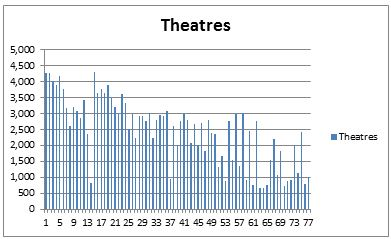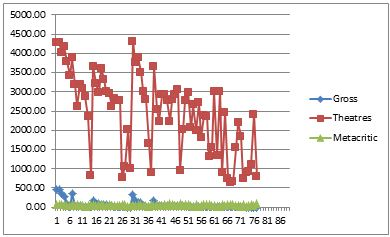## A. Ans

Descriptive statistics of Gross Revenue:

 Column1 Mean 65.92714286 Standard Error 12.74835205 Median 26.82 Mode #N/A Standard Deviation 111.8663352 Sample Variance 12514.07696 Kurtosis 11.76090225 Skewness 3.24359001 Range 651.6 Minimum 0.67 Maximum 652.27 Sum 5076.39 Count 77

This analysis tells that average gross revenue is 65.92. the standard deviation of this distribution is large so we can say that there is more fluctuation in the data.

### Column1

Mean

2460.052

Standard Error

117.8053

Median

2720

Mode

3003

Standard Deviation

1033.737

Sample Variance

1068613

Kurtosis

-0.87365

Skewness

-0.23422

Range

3658

Minimum

653

Maximum

4311

Sum

189424

Count

77

The data of theatres shows that the average value is 3003 and the standard deviation is 1033. It is negatively skewed data from skewness measure which is -0.234.

### Descriptive statistics of MeteoriticScore:

 Column1 Mean 54.71429 Standard Error 2.049445 Median 56 Mode 81 Standard Deviation 17.98381 Sample Variance 323.4173 Kurtosis -0.99233 Skewness 0.061818 Range 67 Minimum 22 Maximum 89 Sum 4213 Count 77

The mean of a meteoritic score is 54.71. it is positively skewed data. Only 16 movies are of the category in a robot. Their average metacritique ranking is 54.66667 which shows that it is very close to the on an average metacritique ranking.

### B. AnsC. Ans

Box-and-whisker plot for the distribution of the gross revenue:

D. Ans

Correlation between a rating of a film and the revenue

0.160167647

They are positively correlated so we can say that both the data is in the same direction. If one is increasing then another will also increase.

### E. Ans

 Column1 Largest(1) 4311 Smallest(1) 653 Confidence Level(90.0%) 196.16365

### F. Ans

No of action films analyses: = 13

Mean of their gross income = 228.38  millions

P value for the liklyhood test = 0.003102892 . so it is very less probable that film earn this much amount.

### G. Ans

Regression output of Y

 SUMMARY OUTPUT Regression Statistics Multiple R 0.781191 R Square 0.61026 Adjusted R Square 0.555433 Standard Error 58.77616 Observations 76 ANOVA df SS MS F Significance F Regression 8 367832.9 45979.11 15.21073 1.81E-12 Residual 68 234915.3 3454.637 Total 76 602748.2 Coefficients Standard Error t Stat P-value Lower 95% Upper 95% Lower 95.0% Upper 95.0% Intercept -159.867 32.93921 -4.85339 7.42E-06 -225.596 -94.1376 -225.596 -94.1376 4291 0.047216 0.00852 5.541532 5.27E-07 0.030214 0.064218 0.030214 0.064218 59 1.354561 0.418749 3.234777 0.001881 0.518959 2.190162 0.518959 2.190162 1 65.2928 20.40998 3.199063 0.002095 24.56532 106.0203 24.56532 106.0203 1 46.23357 26.21074 1.763917 0.082238 -6.06914 98.53627 -6.06914 98.53627 0 13.34658 31.99987 0.417082 0.677932 -50.5082 77.20133 -50.5082 77.20133 0 12.27422 25.26018 0.485912 0.628592 -38.1317 62.68012 -38.1317 62.68012 0 13.32961 23.82147 0.559563 0.577616 -34.2054 60.86461 -34.2054 60.86461 0 0 0 65535 #NUM! 0 0 0 0

#### H. Ans

The regression line found In the previous examples tells the relationship between Y Gross Revenue in Millions of US\$ and X Number of theatres. P value in this test is equal to 5.71E-11. This less than 0.05 so we can say that we need to accept the null hypothesis. The intercept of the coefficient is -93.6278 and the standard error in this 75.2322.

#### I. Ans

The regression line in above case can be written as

Y =  -159.867 + 0.047216 X1 + 1.354561 X2+  65.2928 X3 + 46.23357 X4  +  13.34658 X5  +  12.27422 X6 + 13.32961 X7 + 0 X8

It is a relationship between Gross Revenue in Millions of US\$ and other variables. The gross revenue can be predicted from the number of theaters and other variables. The intercept part is -159.867. it is negative so we can say that in the case where all variables are 0 then it shows the negative gross income so there is a loss in this business. The coefficient of last variable is 0 , it means that it does not affect the gross income.

#### J. Ans

 R Square 0.61026 Adjusted R Square 0.555433

The adjusted R squire is a modified version of R squire. In the prediction of the output we modified the relation and it shows that the updated r squire. If the adjusted R squire is larger than it means that the expected value is larger than the actual one.

K. Ans

Since p value is less than 0.05 in all cases except X3, X4, X5 so we can say that overall modal satisfying the required significance except these variables.

L. Ans

There are eight independent variables which effect the gross revenue in this regression modal but since the coefficient of X8 is 0 so we can say that except thriller all other factors effects the gross profit.

#### M. Ans

It is given that

Y gross profit

X1 Metacritique score of 30
X2    Shows in 4,000 theatres
X3  Not a sequel or a reboot

Regression analysis result

 SUMMARY OUTPUT Regression Statistics Multiple R 0.767026 R Square 0.588329 Adjusted R Square 0.571176 Standard Error 58.70521 Observations 76 ANOVA df SS MS F Significance F Regression 3 354614.5 118204.8 34.29904 7.04E-14 Residual 72 248133.7 3446.302 Total 75 602748.2 Coefficients Standard Error t Stat P-value Lower 95% Upper 95% Lower 95.0% Upper 95.0% Intercept -158.8 30.8676 -5.14457 2.23E-06 -220.334 -97.267 -220.334 -97.267 4291 0.051576 0.007616 6.771887 2.9E-09 0.036393 0.066759 0.036393 0.066759 59 1.447145 0.385132 3.757532 0.000346 0.679399 2.214892 0.679399 2.214892 1 66.64747 19.42855 3.430388 0.001001 27.91736 105.3776 27.91736 105.3776

Regression line is Y = -158.8 + 0.051 X1+1.447 X2+ 66.64 X3

Predicted Y =-158.8 + 0.051 x 30+1.447 x 4000+ 66.64 x 0

Y = 5601.65

#### N. Ans

Following graph shows the connection among these three variables. The curves shows that the nonlinear distribution of Gross profit, the theatres. The graph of metacritique shows the linear distribution or we can say that it is closed to linear distribution.### O. Ans

The above analysis tells very much information about the movies interest Australia. We can see that only 16 movies are of category reboot. On other side 15 movies are of comedy category their average metacritique ranking is 51.66667.  it is very less in the case of metacritique ranking of the movies which are under reboot category. So it directly tells that most of the people taking interest in the movies of reboot category. This interest is larger than the movies of action categories. There are 14 movies are of categories in action so we can see that the average of the metacritique ranking of these movies is 52.71429. it is less than the average ranking.  The average gross income of action movies is 156.37. it is larger than the average income of all type of the movies so we can try to analyze this interest also.